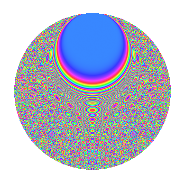# Properties

 Label 363.2.mLevel 363 Weight 2 Character orbit m Rep. character $$\chi_{363}(4,\cdot)$$ Character field $$\Q(\zeta_{55})$$ Dimension 880 Newform subspaces 2 Sturm bound 88 Trace bound 2

# Related objects

## Defining parameters

 Level: $$N$$ = $$363 = 3 \cdot 11^{2}$$ Weight: $$k$$ = $$2$$ Character orbit: $$[\chi]$$ = 363.m (of order $$55$$ and degree $$40$$) Character conductor: $$\operatorname{cond}(\chi)$$ = $$121$$ Character field: $$\Q(\zeta_{55})$$ Newform subspaces: $$2$$ Sturm bound: $$88$$ Trace bound: $$2$$ Distinguishing $$T_p$$: $$2$$

## Dimensions

The following table gives the dimensions of various subspaces of $$M_{2}(363, [\chi])$$.

Total New Old
Modular forms 1840 880 960
Cusp forms 1680 880 800
Eisenstein series 160 0 160

## Trace form

 $$880q + 4q^{2} + 26q^{4} + 4q^{5} + 2q^{6} + 2q^{7} - 8q^{8} - 220q^{9} + O(q^{10})$$ $$880q + 4q^{2} + 26q^{4} + 4q^{5} + 2q^{6} + 2q^{7} - 8q^{8} - 220q^{9} - 26q^{10} - 20q^{11} + 8q^{12} - 42q^{13} - 54q^{14} - 2q^{15} + 2q^{16} - 14q^{17} - 6q^{18} + 20q^{19} - 14q^{20} - 16q^{21} - 40q^{22} - 16q^{23} - 12q^{24} + 20q^{25} - 22q^{26} + 12q^{28} + 4q^{29} - 22q^{31} + 48q^{32} + 10q^{33} + 40q^{34} + 26q^{36} - 76q^{37} + 6q^{38} + 16q^{39} - 136q^{40} - 20q^{41} + 6q^{42} - 16q^{43} - 56q^{44} + 4q^{45} - 10q^{46} - 22q^{47} - 8q^{48} - 36q^{49} - 282q^{50} - 164q^{52} - 146q^{53} - 8q^{54} - 104q^{55} - 70q^{56} - 62q^{58} + 6q^{59} - 12q^{60} + 18q^{61} - 82q^{62} + 2q^{63} - 58q^{64} - 38q^{65} + 12q^{66} - 58q^{67} + 28q^{68} + 2q^{69} - 66q^{70} - 96q^{71} + 2q^{72} - 42q^{73} + 30q^{74} - 16q^{75} - 282q^{76} + 2q^{77} - 78q^{78} - 126q^{79} - 58q^{80} - 220q^{81} - 62q^{82} - 34q^{83} - 86q^{85} - 40q^{86} - 24q^{87} + 18q^{88} - 20q^{89} - 26q^{90} - 104q^{91} - 262q^{92} - 28q^{93} - 370q^{94} - 30q^{95} - 124q^{96} - 60q^{97} - 216q^{98} - 30q^{99} + O(q^{100})$$

## Decomposition of $$S_{2}^{\mathrm{new}}(363, [\chi])$$ into newform subspaces

Label Dim. $$A$$ Field CM Traces $q$-expansion
$$a_2$$ $$a_3$$ $$a_5$$ $$a_7$$
363.2.m.a $$440$$ $$2.899$$ None $$1$$ $$110$$ $$3$$ $$-1$$
363.2.m.b $$440$$ $$2.899$$ None $$3$$ $$-110$$ $$1$$ $$3$$

## Decomposition of $$S_{2}^{\mathrm{old}}(363, [\chi])$$ into lower level spaces

$$S_{2}^{\mathrm{old}}(363, [\chi]) \cong$$ $$S_{2}^{\mathrm{new}}(121, [\chi])$$$$^{\oplus 2}$$

## Hecke Characteristic Polynomials

There are no characteristic polynomials of Hecke operators in the database